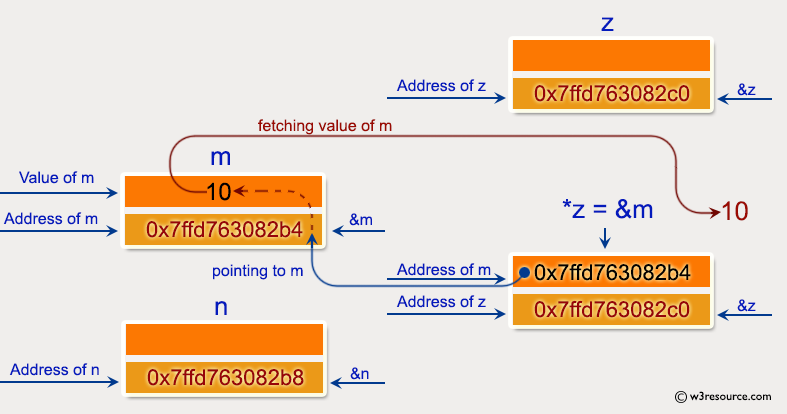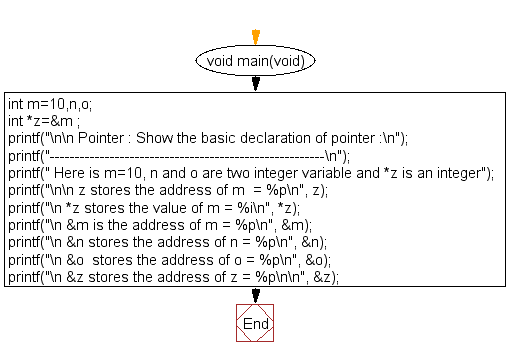﻿ C Program: Show the basic declaration of pointer - w3resource# C Exercises: Show the basic declaration of pointer

## C Pointer : Exercise-1 with Solution

Write a program in C to show the basic declaration of pointer.

Pictorial Presentation:Sample Solution:

C Code:

``````#include <stdio.h>
void main(void)
{
int m=10,n,o;
int *z=&m ;

printf("\n\n Pointer : Show the basic declaration of pointer :\n");
printf("-------------------------------------------------------\n");
printf(" Here is m=10, n and o are two integer variable and *z is an integer");
printf("\n\n z stores the address of m  = %p\n",  z); // z is a pointer so %p would print the address
printf("\n *z stores the value of m = %i\n",   *z);
printf("\n &m is the address of m = %p\n",   &m); // &m gives the address of the integer variable m
// so %p is the specifier for that address
printf("\n &n stores the address of n = %p\n",   &n);
printf("\n &o  stores the address of o = %p\n",   &o);
printf("\n &z stores the address of z = %p\n\n", &z); // &z gives the address, where the pointer z is
// stored -> still an address -> %p is the right
// specifier
}
```
```

Sample Output:

``` Pointer : Show the basic declaration of pointer :
-------------------------------------------------------
Here is m=10, n and o are two integer variable and *z is an integer

z stores the address of m  = 0x7ffd763082b4

*z stores the value of m = 10

&m is the address of m = 0x7ffd763082b4

&n stores the address of n = 0x7ffd763082b8

&o  stores the address of o = 0x7ffd763082bc

&z stores the address of z = 0x7ffd763082c0
```

Flowchart:C Programming Code Editor:

Have another way to solve this solution? Contribute your code (and comments) through Disqus.

What is the difficulty level of this exercise?

Test your Programming skills with w3resource's quiz.

﻿

## C Programming: Tips of the Day

How to format strings using printf() to get equal length in the output?

You can specify a width on string fields, e.g.

`printf("%-20s", "initialization..."); `

And then whatever's printed with that field will be blank-padded to the width you indicate.

The - left-justifies your text in that field.

Ref : https://bit.ly/34DMOc3